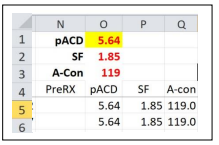top of page
Search

# Kenneth J Hoffer - Zeroing Out the Mean Error in IOL Power Calculation Studies in Excel

Updated: Mar 23, 2022

Kenneth J Hoffer MD

When comparing results of studies on IOL power formulas it is always necessary to Zero out the Mean Error (ME) of each formula. This eliminates the artificial effect of the lens constant in the calculations. It is similar to the method to determine a personalized lens constant for an individual IOL style. As example, let’s say we are testing the Hoffer Q formula which uses pACD as its Lens Constant.STEP 1: In an area at the top of the worksheet (above the rows of calculations for individual subjects) define one individual cell as the Value of the Lens Constant pACD. E.g. Cell O1 to the right in Yellow.

STEP 2: E.g., Enter the starting value of pACD as 5.64 for this IOL.STEP 3: Now the values for pACD in each Row of the patient data must have its numerical value changed to input the value that is in Cell O1. This is done by first typing “+O1” into the first cell, e.g. Cell O5 (in blue).STEP 4: When you click Enter, the formula “+O1” now becomes the value in O1, i.e. “5.64” and the fx box at the top shows the formula “=+O1”.

STEP 5: Now, this formula “+O1” must be copied from Cell O5 and then pasted down the entire column O so that each patient data will now use the value in Cell O1 to perform the calculation of the Prediction Error rather than using the value that was originally entered.STEP 6: Perform all the calculations so that you reach a Mean Prediction Error located in the spreadsheet. E.g. The PE for each eye is calculated in Column X, e.g. So enter the formula to perform an Average of all the PEs in Column X [=AVERAGE(X5:X649)] appear below the last value; e.g. in Cell X650.

STEP 7: Now the Zeroing Out can be performed. In the File Menu at the top, Click on “Data”.Along the upper Menu select the button “What-If Analysis” and you will see 3 choices: Select “Goal Seek” and click on it. A box will open up whereby you need to enter 3 values. “Set Cell” must be set at the Cell holding the ME (e.g. X650); “To Value” must always be set to Zero (0); “By Changing Cell” must be set to the lens constant cell at the top (Cell O1, e.g.)When the MEs for all formulas = 0, it is now valid to compare their SDs, Median Absolute Errors, etc.